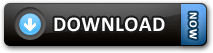### PHP Script to add or subtract days, months, years to a Date!Here is another Interesting PHP script which you can use it in your project. A PHP script to add or subtract days, months and years to date.

Preview:Adding days or months or year to a date in PHP is a piece of cake if you are an experienced PHP person. Just see the snippet below:

```<?php
//this will add a day to the current date
strtotime('+ 1 days');

//this will add a month to the current date
strtotime('+ 1 month');

//this will add a year to the current date
strtotime('+ 1 year');

?>```

Easy right? But I have elaborated the above snippet to a useful PHP script along with HTML and Ajax. You can modify and use it in your projects if you wish to.

HTML and jQuery part (Front end – index.php):

```<html>
<title>PHP Script to add or subtract days, months, year to a Date!</title>
<meta charset="UTF-8">
<meta name="viewport" content="width=device-width, initial-scale=1.0">
<script>
\$(function(){
//on click of the Find new date button
\$('#findNewDate').click(function(){
//send the form data to caldata.php
\$.post('caldate.php', \$('#myform').serialize(), function(res){
//display the result from caldate.php
\$('#result').html(res);
});
});
});
</script>
<body>
<h2>PHP Script to add or subtract days, months, year to a Date!</h2>
<form action="" name="myform" id="myform" method="post">
Select a date:  <input type="date" name="selDate" value="<?php echo date('Y-m-d'); ?>" /><br /><br />
Days <input type="number" min="0" max="10000" value="0" name="days" />&nbsp;
Months <input type="number" min="0" max="10000" value="0" name="months" />&nbsp;
Years <input type="number" min="0" max="10000" value="0" name="years" />&nbsp;
&nbsp;<input type="button" value="Find new date!" id="findNewDate" /><br /><br />
<div id="result"></div>
</form>
</body>
</html>
```

Read the in-line comments in the above program to understand the script.

PHP date calculation script: (Backend – caldate.php):

```<?php
//capture the form data
\$seldate = \$_POST['selDate'];
\$days = \$_POST['days'];
\$months = \$_POST['months'];
\$years = \$_POST['years'];

//in a single line we are adding days, months and years to the \$selDate (selected date)

//ouput the result
print "<b>Selected Date: </b>". date('m-d-Y', strtotime(\$seldate)) . "<br />";
print "<b>New Date: </b>". date('m-d-Y', \$addDays) . "<br />";

?>
```

Above Frontend and Backend script can be written in a single page, but I have implemented Ajax to not to refresh the page after every click of the ‘Find New Date’ button.

And you can download the script from below as a zip file: